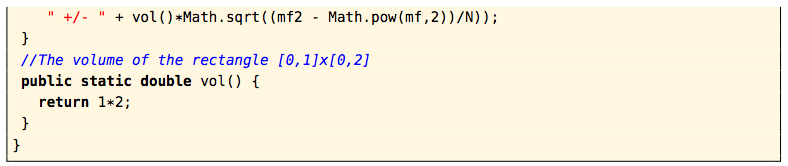$$\newcommand{\id}{\mathrm{id}}$$ $$\newcommand{\Span}{\mathrm{span}}$$ $$\newcommand{\kernel}{\mathrm{null}\,}$$ $$\newcommand{\range}{\mathrm{range}\,}$$ $$\newcommand{\RealPart}{\mathrm{Re}}$$ $$\newcommand{\ImaginaryPart}{\mathrm{Im}}$$ $$\newcommand{\Argument}{\mathrm{Arg}}$$ $$\newcommand{\norm}{\| #1 \|}$$ $$\newcommand{\inner}{\langle #1, #2 \rangle}$$ $$\newcommand{\Span}{\mathrm{span}}$$

# 3.4: Numerical Approximation of Multiple Integrals

•• Contributed by Michael Corral
• Professor (Mathematics) at Schoolcraft College

$$\newcommand{\vecs}{\overset { \rightharpoonup} {\mathbf{#1}} }$$

$$\newcommand{\vecd}{\overset{-\!-\!\rightharpoonup}{\vphantom{a}\smash {#1}}}$$

As you have seen, calculating multiple integrals is tricky even for simple functions and regions. For complicated functions, it may not be possible to evaluate one of the iterated integrals in a simple closed form. Luckily there are numerical methods for approximating the value of a multiple integral. The method we will discuss is called the Monte Carlo method. The idea behind it is based on the concept of the average value of a function, which you learned in single-variable calculus. Recall that for a continuous function $$f (x)$$, the average value $$\bar f \text{ of }f$$ over an interval $$[a,b]$$ is defined as

$\bar f = \dfrac{1}{b-a}\int_a^b f (x)\,dx \label{Eq3.11}$

The quantity $$b − a$$ is the length of the interval $$[a,b]$$, which can be thought of as the “volume” of the interval. Applying the same reasoning to functions of two or three variables, we define the average value of $$f (x, y)$$ over a region $$R$$ to be

$\bar f = \dfrac{1}{A(R)} \iint\limits_R f (x, y)\,d A \label{Eq3.12}$

where $$A(R)$$ is the area of the region $$R$$, and we define the average value of $$f (x, y, z)$$ over a solid $$S$$ to be

$\bar f = \dfrac{1}{V(S)} \iiint\limits_S f (x, y, z)\,dV \label{Eq3.13}$

where $$V(S)$$ is the volume of the solid $$S$$. Thus, for example, we have

$\iint\limits_R f (x, y)\,d A = A(R) \bar f \label{Eq3.14}$

The average value of $$f (x, y)$$ over $$R$$ can be thought of as representing the sum of all the values of $$f$$ divided by the number of points in $$R$$. Unfortunately there are an infinite number (in fact, uncountably many) points in any region, i.e. they can not be listed in a discrete sequence. But what if we took a very large number $$N$$ of random points in the region $$R$$ (which can be generated by a computer) and then took the average of the values of $$f$$ for those points, and used that average as the value of $$\bar f$$ ? This is exactly what the Monte Carlo method does. So in Formula \ref{Eq3.14} the approximation we get is

$\iint\limits_R f (x, y)\,d A \approx A(R) \bar f \pm A(R) \sqrt{\dfrac{\bar {f^2}-(\bar f)^2}{N}}\label{Eq3.15}$

where

$\bar f = \dfrac{\sum_{i=1}^{N}f(x_i,y_i)}{N}\text{ and } \bar {f^2} = \dfrac{\sum_{i=1}^{N}(f(x_i,y_i))^2}{N}\label{Eq3.16}$

with the sums taken over the $$N$$ random points $$(x_1 , y_1), ..., (x_N , y_N )$$. The $$\pm$$ “error term” in Formula \ref{Eq3.15} does not really provide hard bounds on the approximation. It represents a single standard deviation from the expected value of the integral. That is, it provides a likely bound on the error. Due to its use of random points, the Monte Carlo method is an example of a probabilistic method (as opposed to deterministic methods such as Newton’s method, which use a specific formula for generating points).

For example, we can use Formula \ref{Eq3.15} to approximate the volume $$V$$ under the plane $$z = 8x + 6y$$ over the rectangle $$R = [0,1] \times [0,2]$$. In Example 3.1 in Section 3.1, we showed that the actual volume is 20. Below is a code listing (montecarlo.java) for a Java program that calculates the volume, using a number of points $$N$$ that is passed on the command line as a parameter.Listing 3.1 Program listing for montecarlo.java

The results of running this program with various numbers of random points (e.g. java montecarlo 100) are shown below:As you can see, the approximation is fairly good. As $$N \to \infty$$, it can be shown that the Monte Carlo approximation converges to the actual volume (on the order of $$O( \sqrt{N})$$, in computational complexity terminology).

In the above example the region $$R$$ was a rectangle. To use the Monte Carlo method for a nonrectangular (bounded) region $$R$$, only a slight modification is needed. Pick a rectangle $$\tilde R \text{ that encloses }R$$, and generate random points in that rectangle as before. Then use those points in the calculation of $$\bar f$$ only if they are inside $$R$$. There is no need to calculate the area of $$R$$ for Equation \ref{Eq3.15} in this case, since the exclusion of points not inside $$R$$ allows you to use the area of the rectangle $$\tilde R$$ instead, similar to before.

For instance, in Example 3.4 we showed that the volume under the surface $$z = 8x + 6y$$ over the nonrectangular region $$R = {(x, y) : 0 ≤ x ≤ 1, 0 ≤ y ≤ 2x^2 }$$ is 6.4. Since the rectangle $$\tilde R = [0,1] \times [0,2] \text{ contains }R$$, we can use the same program as before, with the only change being a check to see if $$y < 2x^2$$ for a random point $$(x, y) \text{ in }[0,1] \times [0,2]$$. Listing 3.2 below contains the code (montecarlo2.java):Listing 3.2 Program listing for montecarlo2.java

The results of running the program with various numbers of random points (e.g. java montecarlo2 1000) are shown below:To use the Monte Carlo method to evaluate triple integrals, you will need to generate random triples $$(x, y, z)$$ in a parallelepiped, instead of random pairs $$(x, y)$$ in a rectangle, and use the volume of the parallelepiped instead of the area of a rectangle in Equation \ref{Eq3.15} (see Exercise 2). For a more detailed discussion of numerical integration methods, see PRESS et al.